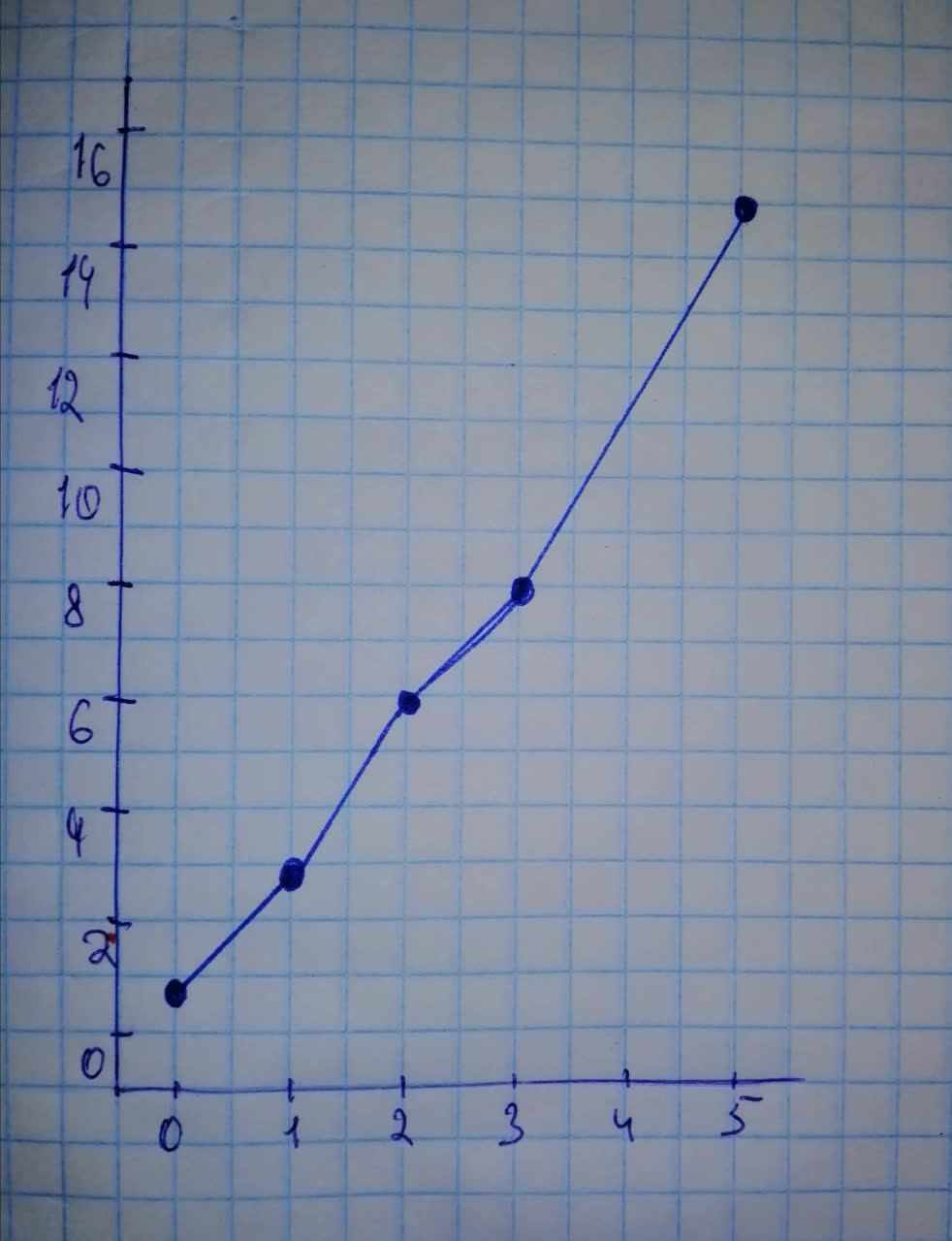# Use a scatterplot and the linear correlation coefficient r to determine whetherClifton Sanchez 2021-11-18 Answered
Use a scatterplot and the linear correlation coefficient r to determine whether there is a correlation between the two variables.
$\begin{array}{|c|c|}\hline x & 1 & 0 & 5 & 2 & 3 \\ \hline y & 3 & 1 & 15 & 6 & 8 \\ \hline \end{array}$

• Questions are typically answered in as fast as 30 minutes

### Plainmath recommends

• Get a detailed answer even on the hardest topics.
• Ask an expert for a step-by-step guidance to learn to do it yourself.Otigh1979
Step 1Step 2
Scatterplot suggest that there is strong linear correlation between the variables $$\displaystyle{r}={0.994}$$
$$\displaystyle{c}{r}{i}{t}{i}{c}{a}{l}-{r}=\pm{878}$$
Since r is more than positive critical value, hence there is a linear correlation between the variables.